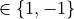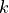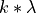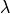# Simulation module#

Module to interact easily with the NEST simulator. It allows to:

## Content#

 nngt.simulation.ActivityRecord(spike_data, ...) Class to record the properties of the simulated activity. nngt.simulation.activity_types(...[, ...]) Analyze the spiking pattern of a neural network. nngt.simulation.analyze_raster([raster, ...]) Return the activity types for a given raster. Get the adjacency matrix describing a NEST network. nngt.simulation.get_recording(network, record) Return the evolution of some recorded values for each neuron. nngt.simulation.make_nest_network(network[, ...]) Create a new network which will be filled with neurons and connector objects to reproduce the topology from the initial network. nngt.simulation.monitor_groups(group_names, ...) Monitoring the activity of nodes in the network. nngt.simulation.monitor_nodes(gids[, ...]) Monitoring the activity of nodes in the network. Plot the monitored activity. Randomize the neural states according to the instructions. nngt.simulation.raster_plot(times, senders) Plotting routine that constructs a raster plot along with an optional histogram. nngt.simulation.reproducible_weights(...[, ...]) Find the values of the connection weights that will give PSP responses of min_weight and max_weight in mV. nngt.simulation.save_spikes(filename[, ...]) Plot the monitored activity. nngt.simulation.set_minis(network, ...[, ...]) Mimick spontaneous release of neurotransmitters, called miniature PSCs or "minis" that can occur at excitatory (mEPSCs) or inhibitory (mIPSCs) synapses. nngt.simulation.set_noise(gids, mean, std) Submit neurons to a current white noise. nngt.simulation.set_poisson_input(gids, rate) Submit neurons to a Poissonian rate of spikes. nngt.simulation.set_step_currents(gids, ...) Set step-current excitations

## Details#

class nngt.simulation.ActivityRecord(spike_data, phases, properties, parameters=None)[source]#

Class to record the properties of the simulated activity.

Initialize the instance using spike_data (store proxy to an optional network) and compute the properties of provided data.

Parameters
• spike_data (2D array) – Array of shape (num_spikes, 2), containing the senders on the 1st row and the times on the 2nd row.

• phases (dict) – Limits of the different phases in the simulated period.

• properties (dict) – Values of the different properties of the activity (e.g. “firing_rate”, “IBI”…).

• parameters (dict, optional (default: None)) – Parameters used to compute the phases.

Note

The firing rate is computed as num_spikes / total simulation time, the period is the sum of an IBI and a bursting period.

nngt.simulation.activity_types(spike_recorder, limits, network=None, phase_coeff=(0.5, 10.0), mbis=0.5, mfb=0.2, mflb=0.05, skip_bursts=0, simplify=False, fignums=[], show=False)[source]#

Analyze the spiking pattern of a neural network.

@todo:

think about inserting t=0. and t=simtime at the beginning and at the end of times.

Parameters
• spike_recorder (NEST node(s) (tuple or list of tuples)) – The recording device that monitored the network’s spikes.

• limits (tuple of floats) – Time limits of the simulation region which should be studied (in ms).

• network (Network, optional (default: None)) – Neural network that was analyzed

• phase_coeff (tuple of floats, optional (default: (0.2, 5.))) – A phase is considered ‘bursting’ when the interspike between all spikes that compose it is smaller than phase_coeff / avg_rate (where avg_rate is the average firing rate), ‘quiescent’ when it is greater that phase_coeff / avg_rate, ‘mixed’ otherwise.

• mbis (float, optional (default: 0.5)) – Maximum interspike interval allowed for two spikes to be considered in the same burst (in ms).

• mfb (float, optional (default: 0.2)) – Minimal fraction of the neurons that should participate for a burst to be validated (i.e. if the interspike is smaller that the limit BUT the number of participating neurons is too small, the phase will be considered as ‘localized’).

• mflb (float, optional (default: 0.05)) – Minimal fraction of the neurons that should participate for a local burst to be validated (i.e. if the interspike is smaller that the limit BUT the number of participating neurons is too small, the phase will be considered as ‘mixed’).

• skip_bursts (int, optional (default: 0)) – Skip the skip_bursts first bursts to consider only the permanent regime.

• simplify (bool, optional (default: False)) – If True, ‘mixed’ phases that are contiguous to a burst are incorporated to it.

• return_steps (bool, optional (default: False)) – If True, a second dictionary, phases_steps will also be returned. @todo: not implemented yet

• fignums (list, optional (default: [])) – Indices of figures on which the periods can be drawn.

• show (bool, optional (default: False)) – Whether the figures should be displayed.

Note

Effects of skip_bursts and limits are cumulative: the limits first milliseconds are ignored, then the skip_bursts first bursts of the remaining activity are ignored.

Returns

phases (dict) – Dictionary containing the time intervals (in ms) for all four phases (bursting’, quiescent’, mixed’, and localized) as lists. E.g: phases["bursting"] could give [[123.5,334.2], [857.1,1000.6]].

nngt.simulation.analyze_raster(raster=None, limits=None, network=None, phase_coeff=(0.5, 10.0), mbis=0.5, mfb=0.2, mflb=0.05, skip_bursts=0, skip_ms=0.0, simplify=False, fignums=[], show=False)[source]#

Return the activity types for a given raster.

Parameters
• raster (array-like (N, 2) or str) – Either an array containing the ids of the spiking neurons on the first column, then the corresponding times on the second column, or the path to a NEST .gdf recording.

• limits (tuple of floats) – Time limits of the simulation regrion which should be studied (in ms).

• network (Network, optional (default: None)) – Network on which the recorded activity was simulated.

• phase_coeff (tuple of floats, optional (default: (0.2, 5.))) – A phase is considered ‘bursting’ when the interspike between all spikes that compose it is smaller than phase_coeff / avg_rate (where avg_rate is the average firing rate), ‘quiescent’ when it is greater that phase_coeff / avg_rate, ‘mixed’ otherwise.

• mbis (float, optional (default: 0.5)) – Maximum interspike interval allowed for two spikes to be considered in the same burst (in ms).

• mfb (float, optional (default: 0.2)) – Minimal fraction of the neurons that should participate for a burst to be validated (i.e. if the interspike is smaller that the limit BUT the number of participating neurons is too small, the phase will be considered as ‘localized’).

• mflb (float, optional (default: 0.05)) – Minimal fraction of the neurons that should participate for a local burst to be validated (i.e. if the interspike is smaller that the limit BUT the number of participating neurons is too small, the phase will be considered as ‘mixed’).

• skip_bursts (int, optional (default: 0)) – Skip the skip_bursts first bursts to consider only the permanent regime.

• simplify (bool, optional (default: False)) – If True, ‘mixed’ phases that are contiguous to a burst are incorporated to it.

• fignums (list, optional (default: [])) – Indices of figures on which the periods can be drawn.

• show (bool, optional (default: False)) – Whether the figures should be displayed.

Note

Effects of skip_bursts and limits are cumulative: the limits first milliseconds are ignored, then the skip_bursts first bursts of the remaining activity are ignored.

Returns

activity (ActivityRecord) – Object containing the phases and the properties of the activity from these phases.

Get the adjacency matrix describing a NEST network.

Parameters

id_converter (dict, optional (default: None)) – A dictionary which maps NEST gids to the desired neurons ids.

Returns

mat_adj (lil_matrix) – Adjacency matrix of the network.

nngt.simulation.get_recording(network, record, recorder=None, nodes=None)[source]#

Return the evolution of some recorded values for each neuron.

Parameters
• network (nngt.Network) – Network for which the activity was simulated.

• record (str or list) – Name of the record(s) to obtain.

• recorder (tuple of ints, optional (default: all multimeters)) – GID of the spike recorder objects recording the network activity.

• nodes (array-like, optional (default: all nodes)) – NNGT ids of the nodes for which the recording should be returned.

Returns

values (dict of dict of arrays) – Dictionary containing, for each record, an M array with the recorded values for n-th neuron is stored under entry n (integer). A times entry is also added; it should be the same size for all records, otherwise an error will be raised.

Examples

After the creation of a Network called net, use the following code:

import nest

rec, _ = monitor_nodes(
net.nest_gids, "multimeter", {"record_from": ["V_m"]}, net)
nest.Simulate(100.)
recording = nngt.simulation.get_recording(net, "V_m")

# access the membrane potential of first neuron + the times
V_m   = recording["V_m"]
times = recording["times"]

nngt.simulation.make_nest_network(network, send_only=None, weights=True)[source]#

Create a new network which will be filled with neurons and connector objects to reproduce the topology from the initial network.

Changed in version 0.8: Added send_only parameter.

Parameters
• network (nngt.Network or nngt.SpatialNetwork) – the network we want to reproduce in NEST.

• send_only (int, str, or list of str, optional (default: None)) – Restrict the nodes that are created in NEST to either inhibitory or excitatory neurons send_onlyto a group or a list of groups.

• weights (bool or str, optional (default: binary edges)) – Whether edge weights should be considered; if None or False then use binary edges; if True, uses the ‘weight’ edge attribute, otherwise uses any valid edge attribute required.

Returns

gids (tuple or NodeCollection (nodes in NEST)) – GIDs of the neurons in the NEST network.

nngt.simulation.monitor_groups(group_names, network, nest_recorder=None, params=None)[source]#

Monitoring the activity of nodes in the network.

Parameters
• group_name (list of strings) – Names of the groups that should be recorded.

• network (Network or subclass) – Network which population will be used to differentiate groups.

• nest_recorder (strings or list, optional (default: spike recorder)) – Device(s) to monitor the network.

• params (dict or list of, optional (default: {})) – Dictionarie(s) containing the parameters for each recorder (see NEST documentation for details).

Returns

• recorders (list or NodeCollection of the recorders’ gids)

• recordables (list of the recordables’ names.)

nngt.simulation.monitor_nodes(gids, nest_recorder=None, params=None, network=None)[source]#

Monitoring the activity of nodes in the network.

Parameters
• gids (tuple of ints or list of tuples) – GIDs of the neurons in the NEST subnetwork; either one list per recorder if they should monitor different neurons or a unique list which will be monitored by all devices.

• nest_recorder (strings or list, optional (default: spike recorder)) – Device(s) to monitor the network.

• params (dict or list of, optional (default: {})) – Dictionarie(s) containing the parameters for each recorder (see NEST documentation for details).

• network (Network or subclass, optional (default: None)) – Network which population will be used to differentiate groups.

Returns

• recorders (list or NodeCollection containing the recorders’ gids)

• recordables (list of the recordables’ names.)

nngt.simulation.plot_activity(gid_recorder=None, record=None, network=None, gids=None, axis=None, show=False, limits=None, histogram=False, title=None, fignum=None, label=None, sort=None, average=False, normalize=1.0, decimate=None, transparent=True, kernel_center=0.0, kernel_std=None, resolution=None, cut_gaussian=5.0, **kwargs)[source]#

Plot the monitored activity.

Changed in version 1.2: Switched hist to histogram and default value to False.

Changed in version 1.0.1: Added axis parameter, restored missing fignum parameter.

Parameters
• gid_recorder (tuple or list of tuples, optional (default: None)) – The gids of the recording devices. If None, then all existing spike_recs are used.

• record (tuple or list, optional (default: None)) – List of the monitored variables for each device. If gid_recorder is None, record can also be None and only spikes are considered.

• network (Network or subclass, optional (default: None)) – Network which activity will be monitored.

• gids (tuple, optional (default: None)) – NEST gids of the neurons which should be monitored.

• axis (matplotlib axis object, optional (default: new one)) – Axis that should be use to plot the activity. This takes precedence over fignum.

• show (bool, optional (default: False)) – Whether to show the plot right away or to wait for the next plt.show().

• histogram (bool, optional (default: False)) – Whether to display the histogram when plotting spikes rasters.

• limits (tuple, optional (default: None)) – Time limits of the plot (if not specified, times of first and last spike for raster plots).

• title (str, optional (default: None)) – Title of the plot.

• fignum (int, or dict, optional (default: None)) – Plot the activity on an existing figure (from figure.number). This parameter is ignored if axis is provided.

• label (str or list, optional (default: None)) – Add labels to the plot (one per recorder).

• sort (str or list, optional (default: None)) – Sort neurons using a topological property (“in-degree”, “out-degree”, “total-degree” or “betweenness”), an activity-related property (“firing_rate” or neuronal property) or a user-defined list of sorted neuron ids. Sorting is performed by increasing value of the sort property from bottom to top inside each group.

• normalize (float or list, optional (default: None)) – Normalize the recorded results by a given float. If a list is provided, there should be one entry per voltmeter or multimeter in the recorders. If the recording was done through monitor_groups, the population can be passed to normalize the data by the nuber of nodes in each group.

• decimate (int or list of ints, optional (default: None)) – Represent only a fraction of the spiking neurons; only one neuron in decimate will be represented (e.g. setting decimate to 5 will lead to only 20% of the neurons being represented). If a list is provided, it must have one entry per NeuralGroup in the population.

• kernel_center (float, optional (default: 0.)) – Temporal shift of the Gaussian kernel, in ms (for the histogram).

• kernel_std (float, optional (default: 0.5% of simulation time)) – Characteristic width of the Gaussian kernel (standard deviation) in ms (for the histogram).

• resolution (float or array, optional (default: 0.1*kernel_std)) – The resolution at which the firing rate values will be computed. Choosing a value smaller than kernel_std is strongly advised. If resolution is an array, it will be considered as the times were the firing rate should be computed (for the histogram).

• cut_gaussian (float, optional (default: 5.)) – Range over which the Gaussian will be computed (for the histogram). By default, we consider the 5-sigma range. Decreasing this value will increase speed at the cost of lower fidelity; increasing it with increase the fidelity at the cost of speed.

• **kwargs (dict) – “color” and “alpha” values can be overriden here.

Warning

Sorting with “firing_rate” only works if NEST gids form a continuous integer range.

Returns

lines (list of lists of matplotlib.lines.Line2D) – Lines containing the data that was plotted, grouped by figure.

nngt.simulation.randomize_neural_states(network, instructions, groups=None, nodes=None, make_nest=False)[source]#

Randomize the neural states according to the instructions.

Changed in version 0.8: Changed ids to nodes argument.

Parameters
• network (Network subclass instance) – Network that will be simulated.

• instructions (dict) – Variables to initialize. Allowed keys are “V_m” and “w”. Values are 3-tuples of type ("distrib_name", double, double).

• groups (list of NeuralGroup, optional (default: None)) – If provided, only the neurons belonging to these groups will have their properties randomized.

• nodes (array-like, optional (default: all neurons)) – NNGT ids of the neurons that will have their status randomized.

• make_nest (bool, optional (default: False)) – If True and network has not been converted to NEST, automatically generate the network, else raises an exception.

Example

instructions = {
"V_m": ("uniform", -80., -60.),
"w": ("normal", 50., 5.)
}

nngt.simulation.raster_plot(times, senders, limits=None, title='Spike raster', histogram=False, num_bins=1000, color='b', decimate=None, axis=None, fignum=None, label=None, show=True, sort=None, sort_attribute=None, network=None, transparent=True, kernel_center=0.0, kernel_std=30.0, resolution=None, cut_gaussian=5.0, **kwargs)[source]#

Plotting routine that constructs a raster plot along with an optional histogram.

Changed in version 1.2: Switched hist to histogram.

Changed in version 1.0.1: Added axis parameter.

Parameters
• times (list or numpy.ndarray) – Spike times.

• senders (list or numpy.ndarray) – Index for the spiking neuron for each time in times.

• limits (tuple, optional (default: None)) – Time limits of the plot (if not specified, times of first and last spike).

• title (string, optional (default: ‘Spike raster’)) – Title of the raster plot.

• histogram (bool, optional (default: True)) – Whether to plot the raster’s histogram.

• num_bins (int, optional (default: 1000)) – Number of bins for the histogram.

• color (string or float, optional (default: ‘b’)) – Color of the plot lines and markers.

• decimate (int, optional (default: None)) – Represent only a fraction of the spiking neurons; only one neuron in decimate will be represented (e.g. setting decimate to 10 will lead to only 10% of the neurons being represented).

• axis (matplotlib axis object, optional (default: new one)) – Axis that should be use to plot the activity.

• fignum (int, optional (default: None)) – Id of another raster plot to which the new data should be added.

• label (str, optional (default: None)) – Label the current data.

• show (bool, optional (default: True)) – Whether to show the plot right away or to wait for the next plt.show().

• kernel_center (float, optional (default: 0.)) – Temporal shift of the Gaussian kernel, in ms.

• kernel_std (float, optional (default: 30.)) – Characteristic width of the Gaussian kernel (standard deviation) in ms.

• resolution (float or array, optional (default: 0.1*kernel_std)) – The resolution at which the firing rate values will be computed. Choosing a value smaller than kernel_std is strongly advised. If resolution is an array, it will be considered as the times were the firing rate should be computed.

• cut_gaussian (float, optional (default: 5.)) – Range over which the Gaussian will be computed (for the histogram). By default, we consider the 5-sigma range. Decreasing this value will increase speed at the cost of lower fidelity; increasing it with increase the fidelity at the cost of speed.

Returns

lines (list of matplotlib.lines.Line2D) – Lines containing the data that was plotted.

nngt.simulation.reproducible_weights(weights, neuron_model, di_param={}, timestep=0.05, simtime=50.0, num_bins=1000, log=False)[source]#

Find the values of the connection weights that will give PSP responses of min_weight and max_weight in mV.

Parameters
• weights (list of floats) – Exact desired synaptic weights.

• neuron_model (string) – Name of the model used.

• di_param (dict, optional (default: {})) – Parameters of the model, default parameters if not supplied.

• timestep (float, optional (default: 0.01)) – Timestep of the simulation in ms.

• simtime (float, optional (default: 10.)) – Simulation time in ms (default: 10).

• num_bins (int, optional (default: 10000)) – Number of bins used to discretize the exact synaptic weights.

• log (bool, optional (default: False)) – Whether bins should use a logarithmic scale.

Note

If the parameters used are not the default ones, they MUST be provided, otherwise the resulting weights will likely be WRONG.

nngt.simulation.save_spikes(filename, recorder=None, network=None, save_positions=True, **kwargs)[source]#

Plot the monitored activity.

New in version 0.7.

Parameters
• filename (str) – Path to the file where the activity should be saved.

• recorder (tuple or list of tuples, optional (default: None)) – The NEST gids of the recording devices. If None, then all existing spike recorders are used.

• network (Network or subclass, optional (default: None)) – Network which activity will be monitored.

• save_positions (bool, optional (default: True)) – Whether to include the position of the neurons in the file; this requires network to be provided.

• **kwargs (see numpy.savetxt())

nngt.simulation.set_minis(network, base_rate, weight, syn_type=1, nodes=None, gids=None)[source]#

Mimick spontaneous release of neurotransmitters, called miniature PSCs or “minis” that can occur at excitatory (mEPSCs) or inhibitory (mIPSCs) synapses. These minis consists in only a fraction of the usual strength of a spike- triggered PSC and can be modeled by a Poisson process. This Poisson process occurs independently at every synapse of a neuron, so a neuron receivinginputs will be subjected to these events with a rate, whereis the base rate.

Parameters
• network (Network object) – Network on which the minis should be simulated.

• base_rate (float) – Rate for the Poisson process on one synapse (), in Hz.

• weight (float or array of size N) – Amplitude of a minitature post-synaptic event.

• syn_type (int, optional (default: 1)) – Synaptic type of the noisy connections. By default, mEPSCs are generated, by taking into account only the excitatory degrees and synaptic weights. To setup mIPSCs, used syn_type=-1.

• nodes (array-like (size N), optional (default: all nodes)) – NNGT ids of the neurons that should be subjected to minis.

• gids (array-like (size N), optional (default: all neurons)) – NEST gids of the neurons that should be subjected to minis.

Note

nodes and gids are not compatible, only one one the two arguments can be used in any given call to set_minis.

nngt.simulation.set_noise(gids, mean, std)[source]#

Submit neurons to a current white noise.

Parameters
• gids (tuple) – NEST gids of the target neurons.

• mean (float) – Mean current value.

• std (float) – Standard deviation of the current

Returns

noise (tuple) – The NEST gid of the noise_generator.

nngt.simulation.set_poisson_input(gids, rate, syn_spec=None, **kwargs)[source]#

Submit neurons to a Poissonian rate of spikes.

Changed in version 2.0: Added kwargs.

Parameters
• gids (tuple) – NEST gids of the target neurons.

• rate (float) – Rate of the spike train (in Hz).

• syn_spec (dict, optional (default: static synapse with weight 1)) – Properties of the connection between the poisson_generator object and the target neurons.

• **kwargs (dict) – Other optional parameters for the poisson_generator.

Returns

poisson_input (tuple) – The NEST gid of the poisson_generator.

nngt.simulation.set_step_currents(gids, times, currents)[source]#

Set step-current excitations

Parameters
• gids (tuple) – NEST gids of the target neurons.

• times (list or numpy.ndarray) – List of the times where the current will change (by default the current generator is initiated at I=0. for t=0.)

• currents (list or numpy.ndarray`) – List of the new current value after the associated time value in times.

Returns

noise (tuple) – The NEST gid of the noise_generator.